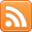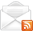# ASP.NET,C#.NET,VB.NET,JQuery,JavaScript,Gridview

aspdotnet-suresh offers C#.net articles and tutorials,csharp dot net,asp.net articles and tutorials,VB.NET Articles,Gridview articles,code examples of asp.net 2.0 /3.5,AJAX,SQL Server Articles,examples of .net technologies

### Asp.net Convert Rupees (Numbers) to Words (String) using C#.NET

Feb 19, 2015
Introduction:

Here I will explain how to convert currency to words in
asp.net using c# or convert numbers to words in asp.net using c# with example or convert currency / numbers to string in using c#.

Description:

In previous post I explained
jQuery tag cloud example in asp.net, sitemap control example, send html page as mail body in asp.net, send mail with images using gmail user credentials, send multiple attachments with email in asp.net, how to send mail with attachment in asp.net and many more articles related to asp.net using c#, vb.net. Now I will explain how to convert currency / numbers to words / string in asp.net using c#, vb.net with example.

To convert currency / numbers to words / string in asp.net using c#, vb.net we need to write the code like as shown below

 C# - Convert Number to Words in Asp.net
Enter Value:

After completion of aspx page write the following code in codebehind

C# Code

 using System; public partial class _Default : System.Web.UI.Page { protected void btnClick_Click(object sender, EventArgs e) { string word = ConvertNumbertoWords(Convert.ToInt32(txtnumber.Text)); lblmsg.InnerText = word; } public static string ConvertNumbertoWords(int number) { if (number == 0) return "ZERO"; if (number < 0) return "minus " + ConvertNumbertoWords(Math.Abs(number)); string words = ""; if ((number / 1000000000) > 0) { words += ConvertNumbertoWords(number / 1000000000) + " Billion "; number %= 1000000000; } if ((number / 10000000) > 0) { words += ConvertNumbertoWords(number / 10000000) + " Crore "; number %= 10000000; } if ((number / 1000000) > 0) { words += ConvertNumbertoWords(number / 1000000) + " MILLION "; number %= 1000000; } if ((number / 1000) > 0) { words += ConvertNumbertoWords(number / 1000) + " THOUSAND "; number %= 1000; } if ((number / 100) > 0) { words += ConvertNumbertoWords(number / 100) + " HUNDRED "; number %= 100; } if (number > 0) { if (words != "") words += "AND "; var unitsMap = new[] { "ZERO", "ONE", "TWO", "THREE", "FOUR", "FIVE", "SIX", "SEVEN", "EIGHT", "NINE", "TEN", "ELEVEN", "TWELVE", "THIRTEEN", "FOURTEEN", "FIFTEEN", "SIXTEEN", "SEVENTEEN", "EIGHTEEN", "NINETEEN" }; var tensMap = new[] { "ZERO", "TEN", "TWENTY", "THIRTY", "FORTY", "FIFTY", "SIXTY", "SEVENTY", "EIGHTY", "NINETY" }; if (number < 20) words += unitsMap[number]; else { words += tensMap[number / 10]; if ((number % 10) > 0) words += " " + unitsMap[number % 10]; } } return words; }
Demo

 If you enjoyed this post, please support the blog below. It's FREE! Get the latest Asp.net, C#.net, VB.NET, jQuery, Plugins & Code Snippets for FREE by subscribing to our Facebook, Twitter, RSS feed, or by email.Subscribe by RSSSubscribe by EmailAnonymous said...

not proper output for value is >10000
if value = 10,000 then output = TEN THOUSAND
if value = 99999 then output = NINETY NINE THOUSAND NINE HUNDRED AND NINETY NINE
now problem is if value is >99999 then output is not proper like
if value = 1,00,000 then output = ONE HUNDRED THOUSAND instead of ONE LAKH of 1 LAKH

thank you.!

shiva said...

Its is working fine , to convert it into lakh ..need to do few changes..thank you

Unknown said...

if i click 20 thousand then display twenty thousands but appears twenty thousand missing "s" so what is the code of that "s".

Unknown said...

but i want lakhs but it show lakh example 2000000 then show twenty lakh .

Amar Singh said...

hi sir,
I am facing the issue that string is not correct format